Share

# RD Sharma solutions for Mathematics for Class 9 chapter 10 - Lines and Angles [Latest edition]

Course
Textbook page

#### Chapters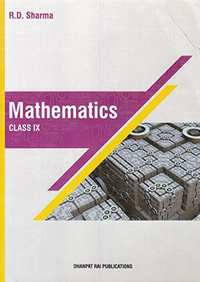## Chapter 10: Lines and Angles

Ex. 10.1Ex. 10.2Ex. 10.3Ex. 10.4Others

#### RD Sharma solutions for Mathematics for Class 9 Chapter 10 Lines and AnglesExercise 10.1 [Page 7]

Ex. 10.1 | Q 1.1 | Page 7

Write the complement of the following angle.

20°

Ex. 10.1 | Q 1.2 | Page 7

Write the complement  of the following angles .

35°

Ex. 10.1 | Q 1.3 | Page 7

Write the complement  of the following angles.

90°

Ex. 10.1 | Q 1.4 | Page 7

Write the complement of the following angles.

77°

Ex. 10.1 | Q 1.5 | Page 7

Write the complement of the following angles .

30°

Ex. 10.1 | Q 2.1 | Page 7

Write the supplement of the following angles .

54°

Ex. 10.1 | Q 2.2 | Page 7

Write the supplement of the following angles.

132°

Ex. 10.1 | Q 2.3 | Page 7

Write the supplement  of the following angles .

138°

Ex. 10.1 | Q 3 | Page 7

If an angle is 28° less than its complement, find its measure.

Ex. 10.1 | Q 4 | Page 7

If an angle is 30° more than one half of its complement, find the measure of the angle.

Ex. 10.1 | Q 5 | Page 7

Two supplementary angles are in the ratio 4 : 5. Find the angles.

Ex. 10.1 | Q 6 | Page 7

Two supplementary angles differ by 48°. Find the angles.

Ex. 10.1 | Q 7 | Page 7

An angle is equal to 8 times its complement. Determine its measure.

Ex. 10.1 | Q 8 | Page 7

If the angles (2x −10)° and (x − 5)° are complementary angles, find x.

Ex. 10.1 | Q 9 | Page 7

If an angle differs from its complement by 10°, find the angle .

Ex. 10.1 | Q 10 | Page 7

If the supplement of an angle is two-third of itself. Determine the angle and its supplement.

Ex. 10.1 | Q 11 | Page 7

An angle is 14° more than its complementary angle. What is its measure?

Ex. 10.1 | Q 12 | Page 7

The measure of an angle is twice the measure of its supplementary ang Find its measure.

Ex. 10.1 | Q 13 | Page 7

If the complement of an angle is equal to the supplement of the thrice of it. Find the measure of the angle.

Ex. 10.1 | Q 14 | Page 7

If the supplement of an angle is three times its complement, find the angle.

#### RD Sharma solutions for Mathematics for Class 9 Chapter 10 Lines and AnglesExercise 10.2 [Pages 14 - 17]

Ex. 10.2 | Q 1.1 | Page 14

In the below Fig, OA and OB are opposite rays :

If x = 25°, what is the value of y?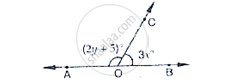Ex. 10.2 | Q 1.2 | Page 14

In the below Fig, OA and OB are opposite rays.

If y = 35°, what is the value of x?Ex. 10.2 | Q 2 | Page 14

In the below fig, write all pairs of adjacent angles and all the linear pairs .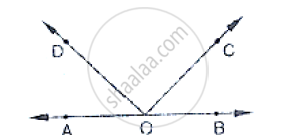Ex. 10.2 | Q 3 | Page 14

In the given below Fig, find x. Further find ∠BOC, ∠COD and ∠AOD .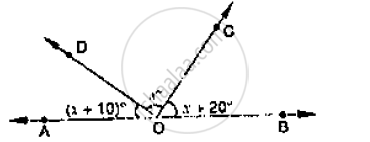Ex. 10.2 | Q 4 | Page 14

In the given below fig, rays OA, OB, OC, OP and 0E have the common end point O. Show
that ∠AOB + ∠BOC + ∠COD + ∠DOE + ∠EOA = 360°.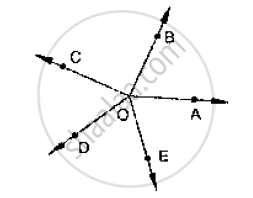Ex. 10.2 | Q 5 | Page 15

In the below Fig, ∠AOC and ∠BOC form a linear pair. if a − 2b = 30°, find a and b.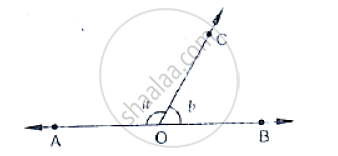Ex. 10.2 | Q 6 | Page 15

How many pairs of adjacent angles are formed when two lines intersect in a point?

Ex. 10.2 | Q 7 | Page 15

How many pairs of adjacent angles, in all, can you name in below fig.?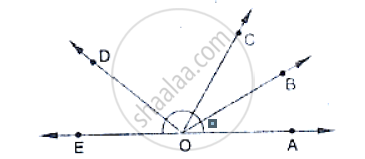Ex. 10.2 | Q 8 | Page 15

In below fig, determine the value of x.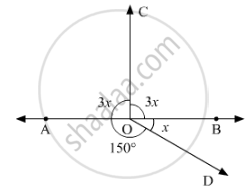Ex. 10.2 | Q 9 | Page 15

In the below fig, AOC is a line, find x.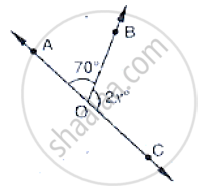Ex. 10.2 | Q 10 | Page 15

In the below fig, POS is a line, find x.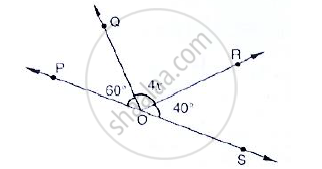Ex. 10.2 | Q 11 | Page 15

In the below fig, ACB is a line such that ∠DCA = 5x and ∠DCB = 4x. Find the value of x.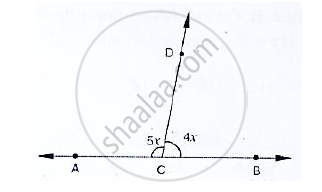Ex. 10.2 | Q 12 | Page 15

Given ∠POR = 3x and ∠QOR = 2x + 10, find the value of x for which POQ will be a line.
(Below fig).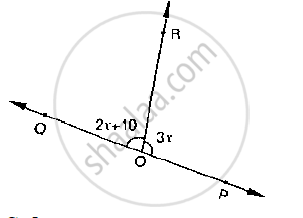Ex. 10.2 | Q 13 | Page 15

What value of y would make AOB a line in below fig, if ∠AOC = 4y and ∠BOC = (6y +
30)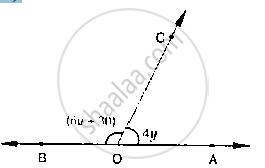Ex. 10.2 | Q 14 | Page 16

In below fig, OP, OQ, OR and OS arc four rays. Prove that:
∠POQ + ∠QOR + ∠SOR + ∠POS = 360°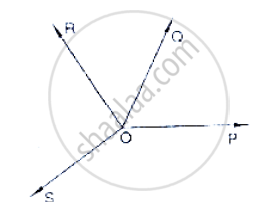Ex. 10.2 | Q 15 | Page 16

In below fig, ray OS stand on a line POQ. Ray OR and ray OT are angle bisectors of ∠POS
and ∠SOQ respectively. If ∠POS = x, find ∠ROT.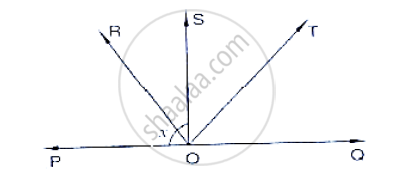Ex. 10.2 | Q 16 | Page 16

In the below fig, lines PQ and RS intersect each other at point O. If ∠POR: ∠ROQ − 5 : 7,
find all the angles.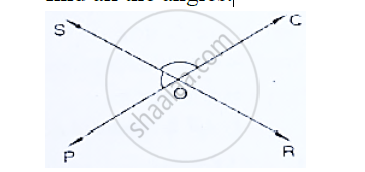Ex. 10.2 | Q 17 | Page 16

In Fig. 8.42, a is greater than b by one third of a right-angle. Find the values of a and b.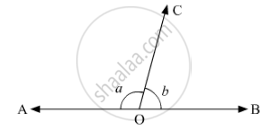Ex. 10.2 | Q 18 | Page 16

If below fig, ∠AOF and ∠FOG form a linear pair.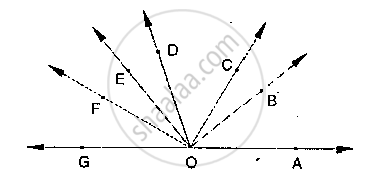∠EOB = ∠FOC = 90° and ∠DOC = ∠FOG = ∠AOB = 30°
(i) Find the measures of ∠FOE, ∠COB and ∠DOE.
(ii) Name all the right angles.
(iii) Name three pairs of adjacent complementary angles.
(iv) Name three pairs of adjacent supplementary angles.
(v) Name three pairs of adjacent angles.

Ex. 10.2 | Q 19 | Page 17

In the below fig, POQ is a line. Ray OR is perpendicular to line OS is another ray lying

between rays OP and OR. Prove that ∠ROS = 1 (∠QOS − ∠POS).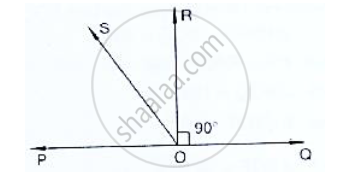#### RD Sharma solutions for Mathematics for Class 9 Chapter 10 Lines and AnglesExercise 10.3 [Pages 22 - 23]

Ex. 10.3 | Q 1 | Page 22

In the below fig, lines �1 and �2 intersect at O, forming angles as shown in the figure. If x = 45, Find the values of x, y, z and u.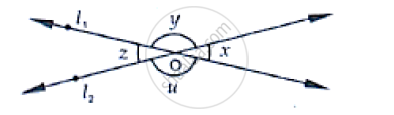Ex. 10.3 | Q 2 | Page 22

In the below fig, three coplanar lines intersect at a point O, forming angles as shown in the figure. Find the values of x, y, z and u.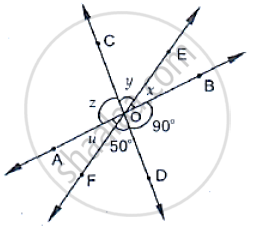Ex. 10.3 | Q 3 | Page 22

In the given fig, find the values of x, y and z.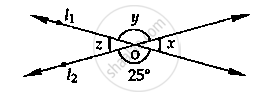Ex. 10.3 | Q 4 | Page 22

In the below fig, find the value of x.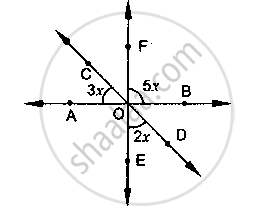Ex. 10.3 | Q 5 | Page 22

If one of the four angles formed by two intersecting lines is a right angle, then show that
each of the four angles is a right angle.

Ex. 10.3 | Q 6.1 | Page 23

In the below fig, rays AB and CD intersect at O.
Determine y when x = 60°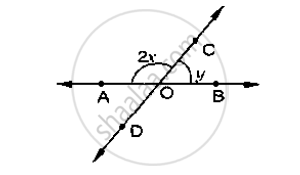Ex. 10.3 | Q 6.2 | Page 23

In the below fig, rays AB and CD intersect at O

Determine x when y =40Ex. 10.3 | Q 7 | Page 23

In the below fig, lines AB, CD and EF intersect at O. Find the measures of ∠AOC, ∠COF,
∠DOE and ∠BOF.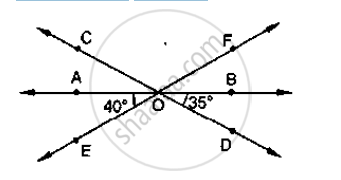Ex. 10.3 | Q 8 | Page 23

AB, CD and EF are three concurrent lines passing through the point O such that OF bisects
∠BOD. If ∠BOF = 35°, find ∠BOC and ∠AOD.

Ex. 10.3 | Q 9 | Page 23

In below figure, lines AB and CD intersect at O. If ∠AOC + ∠BOE = 70° and ∠BOD =
40°, find ∠BOE and reflex ∠COE.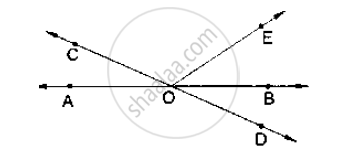Ex. 10.3 | Q 10.1 | Page 23

statement are true and false
Angles forming a linear pair are supplementary.

Ex. 10.3 | Q 10.2 | Page 23

statement are true and false

If two adjacent angles are equal, and then each angle measures 90°.

Ex. 10.3 | Q 10.3 | Page 23

statement are true and false

Angles forming a linear pair can both the acute angles.

Ex. 10.3 | Q 10.4 | Page 23

statement are true and false

If angles forming a linear pair are equal, then each of these angles is of measure 90°.

Ex. 10.3 | Q 11.1 | Page 23

Fill in the blank so as to make the following statement true:

If one angle of a linear pair is acute, then its other angle will be _____

Ex. 10.3 | Q 11.2 | Page 23

Fill in the blank so as to make the following statement true:

A ray stands on a line, then the sum of the two adjacent angles so formed is ______

Ex. 10.3 | Q 11.3 | Page 23

Fill in the blank so as to make the following statement true:

If the sum of two adjacent angles is 180°, then the ______ arms of the two angles are
opposite rays

Ex. 10.3 | Q 12 | Page 23

Prove that the bisectors of a pair of vertically opposite angles are in the same straight line.

Ex. 10.3 | Q 13 | Page 23

If two straight lines intersect each other, prove that the ray opposite to the bisector of one of the angles thus formed bisects the vertically opposite angle.

#### RD Sharma solutions for Mathematics for Class 9 Chapter 10 Lines and AnglesExercise 10.4 [Pages 46 - 49]

Ex. 10.4 | Q 1 | Page 46

In below fig, AB CD and ∠1 and ∠2 are in the ratio 3 : 2. Determine all angles from 1 to 8.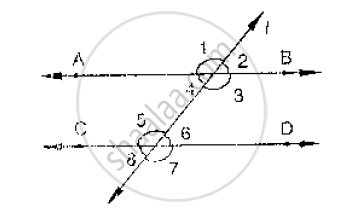Ex. 10.4 | Q 2 | Page 46

In the below fig, l, m and n are parallel lines intersected by transversal p at X, Y and Z
respectively. Find ∠1, ∠2 and ∠3.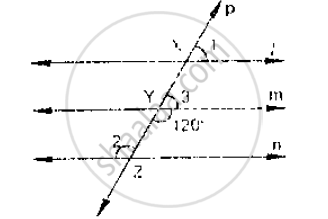Ex. 10.4 | Q 3 | Page 46

If below fig, if AB || CD and CD || EF, find ∠ACE.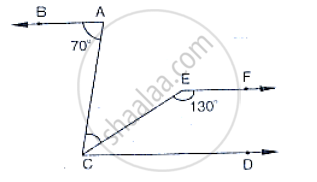Ex. 10.4 | Q 4 | Page 46

In the below fig, state which lines are parallel and why?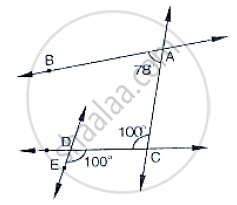Ex. 10.4 | Q 5 | Page 47

In the below fig, if l || m, n || p and ∠1 = 85°, find ∠2.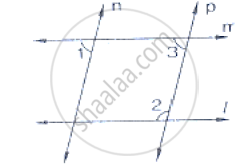Ex. 10.4 | Q 6 | Page 47

If two straight lines are perpendicular to the same line, prove that they are parallel to each
other.

Ex. 10.4 | Q 7 | Page 47

Two unequal angles of a parallelogram are in the ratio 2 : 3. Find all its angles in degrees .

Ex. 10.4 | Q 8 | Page 47

If each of the two lines is perpendicular to the same line, what kind of lines are they to each
other?

Ex. 10.4 | Q 9 | Page 47

In the below fig, ∠1 = 60° and  ∠2 = (2/3)^(rd)of a right angle. Prove that l || m.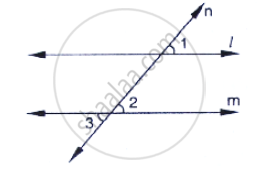Ex. 10.4 | Q 10 | Page 47

In the below fig, if l || m || n and ∠1 = 60°, find ∠2.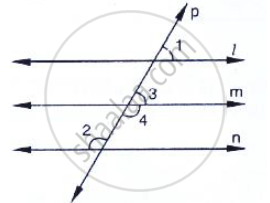Ex. 10.4 | Q 11 | Page 47

Prove that the straight lines perpendicular to the same straight line are parallel to one
another.

Ex. 10.4 | Q 12 | Page 47

The opposite sides of a quadrilateral are parallel. If one angle of the quadrilateral is 60°,
find the other angles.

Ex. 10.4 | Q 13 | Page 47

Two lines AB and CD intersect at O. If ∠AOC + ∠COB + ∠BOD = 270°, find the
measures of ∠AOC, ∠COB, ∠BOD and ∠DOA.

Ex. 10.4 | Q 14 | Page 47

In the below fig, p is a transversal to lines m and n,∠2 = 120° and a∠5 = 60°. Prove that m || n.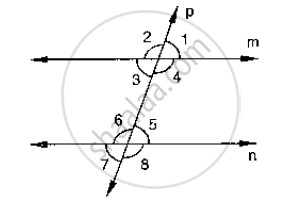Ex. 10.4 | Q 15 | Page 47

In the below fig, transversal 𝑙 intersects two lines m and n, ∠4 = 110° and ∠7 = 65°. Is m || n?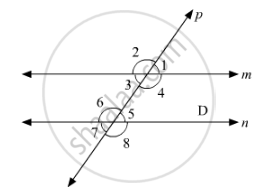Ex. 10.4 | Q 16 | Page 48

Which pair of lines in the below fig, is parallel? Given reasons.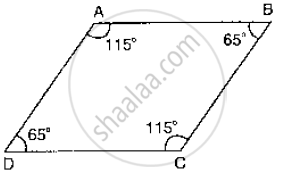Ex. 10.4 | Q 17 | Page 48

If l, m, n are three lines such that l || m and n ⊥ l, prove that n ⊥ m.

Ex. 10.4 | Q 18.1 | Page 48

Which of the following statement are true  and false ? Give reason.

If two lines are intersected by a transversal, then corresponding angles are equal.

Ex. 10.4 | Q 18.2 | Page 48

Which of the following statement are true  and false ? Give reason.

If two parallel lines are intersected by a transversal, then alternate interior angles are equal.

Ex. 10.4 | Q 18.3 | Page 48

Which of the following statement are true  and false ? Give reason.

Two lines perpendicular to the same line are perpendicular to each other.

Ex. 10.4 | Q 18.4 | Page 48

Which of the following statement are true  and false ? Give reason.

Two lines parallel to the same line are parallel to each other.

Ex. 10.4 | Q 18.5 | Page 48

Which of the following statement are true  and false ? Give reason.

If two parallel lines are intersected by a transversal, then the interior angles on the same side of the transversal are equal.

Ex. 10.4 | Q 19.1 | Page 48

Fill in the blank in the following to make the statement true:

If two parallel lines are intersected by a transversal, then each pair of corresponding
angles are _______

Ex. 10.4 | Q 19.2 | Page 48

Fill in the blank in the following to make the statement true:

If two parallel lines are intersected by a transversal, then interior angles on the same
side of the transversal are _______

Ex. 10.4 | Q 19.3 | Page 48

Fill in the blank in the following to make the statement true:

Two lines perpendicular to the same line are _______ to each other.

Ex. 10.4 | Q 19.4 | Page 48

Fill in the blank in the following to make the statement true:

Two lines parallel to the same line are _______ to each other.

Ex. 10.4 | Q 19.5 | Page 48

Fill in the blank in the following to make the statement true:

If a transversal intersects a pair of lines in such a way that a pair of alternate angles
are equal, then the lines are _______

Ex. 10.4 | Q 19.6 | Page 48

Fill in the blank :

If a transversal intersects a pair of lines in such a way that the sum of interior angles
on the same side of transversal is 180°, then the lines are _______.

Ex. 10.4 | Q 20 | Page 48

In the below fig, AB || CD || EF and GH || KL. Find ∠HKL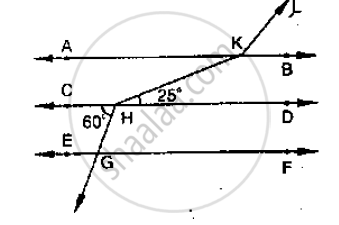Ex. 10.4 | Q 21 | Page 48

In the below fig, show that AB || EF.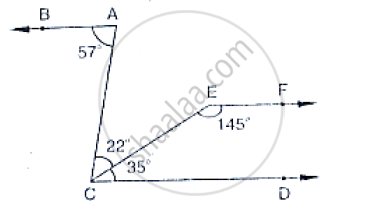Ex. 10.4 | Q 22 | Page 49

In the below , PQ || AB and PR || BC. If ∠QPR = 102°, determine ∠ABC. Give reasons.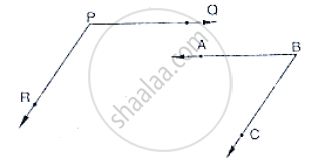Ex. 10.4 | Q 23 | Page 49

Prove that if the two arms of an angle are perpendicular to the two arms of another angle, then the angles are either equal or supplementary

Ex. 10.4 | Q 24 | Page 49

In the below fig, lines AB and CD are parallel and P is any point as shown in the figure.
Show that ∠ABP + ∠CDP = ∠DPB.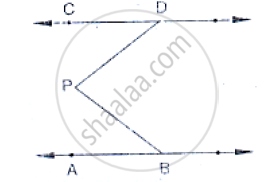Ex. 10.4 | Q 25 | Page 49

In the below fig, AB || CD and P is any point shown in the figure. Prove that:
∠ABP+∠BPD+∠CDP = 36O°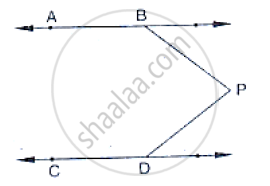Ex. 10.4 | Q 26 | Page 49

In the below fig, arms BA and BC of ∠ABC are respectively parallel to arms ED and EF of
∠DEF. Prove that ∠ABC = ∠DEF.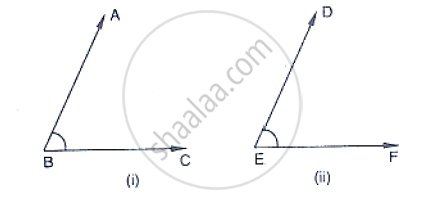Ex. 10.4 | Q 27 | Page 49

In the below fig, arms BA and BC of ∠ABC are respectively parallel to arms ED and EF of
∠DEF. Prove that ∠ABC + ∠DEF = 180°.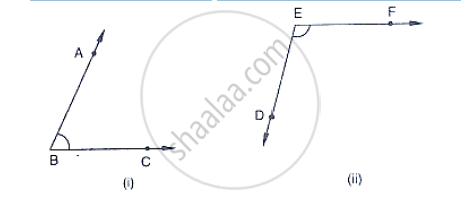#### RD Sharma solutions for Mathematics for Class 9 Chapter 10 Lines and Angles [Page 50]

Q 1 | Page 50

Define complementary angles.

Q 2 | Page 50

Define supplementary angles.

Q 3 | Page 50

Q 4 | Page 50

The complement of an acute angle is ..............

Q 5 | Page 50

The supplement of an acute angle is .................

Q 6 | Page 50

The supplement of a right angle is ..............

Q 7 | Page 50

Write the complement of an angle of measure x°.

Q 8 | Page 50

Write the supplement of an angle of measure 2y°.

Q 9 | Page 50

If a wheel has six spokes equally spaced, then find the measure of the angle between two adjacent spokes.

Q 10 | Page 50

An angle is equal to its supplement. Determine its measure.

Q 11 | Page 50

An angle is equal to five times its complement. Determine its measure.

Q 12 | Page 50

How many pairs of adjacent angles are formed when two lines intersect in a point?

#### RD Sharma solutions for Mathematics for Class 9 Chapter 10 Lines and Angles [Pages 51 - 57]

Q 1 | Page 51

One angle is equal to three times its supplement. The measure of the angle is

• 130°

• 135°

• 90°

• 120°

Q 2 | Page 51

Two straight line AB and CD intersect one another at the point O. If ∠AOC + ∠COB + ∠BOD = 274°, then ∠AOD =

•  86°

•  90°

•  94°

• 137°

Q 3 | Page 51

Two straight lines AB and CD cut each other at O. If ∠BOD = 63°, then ∠BOC =

•  63°

• 117°

•  17°

• 153°

Q 4 | Page 51

Consider the following statements:
When two straight lines intersect:
(iii) opposite angles are equal
(iv) opposite angles are supplementary

Of these statements

•  (i) and (ii) are correct

• (ii) and (iii) are correct

•  (i) and (iv) are correct

• (ii) and (iv)  are correct

Q 5 | Page 51

Given ∠POR = 3x and ∠QOR = 2x + 10°. If POQ is a straight line, then the value of x is

• 30°

• 34°

• 36°

• none of these

Q 6 | Page 51

In the given figure, AOB is a straight line. If ∠AOC + ∠BOD = 85°, then ∠COD =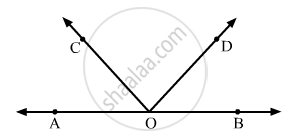• 85°

• 90°

• 95°

• 100°

Q 7 | Page 51

In the given figure, the value of y is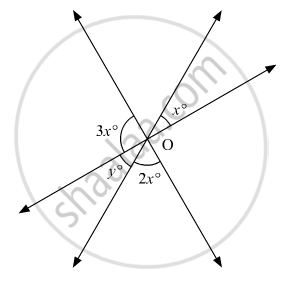• 20°

• 30°

• 45°

• 60°

Q 8 | Page 52

In the given figure, the value of x is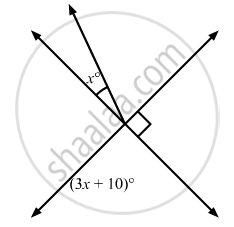•  12

•  15

•  20

•  30

Q 9 | Page 52

In the given figure, which of the following statements must be true?
(i) a + b = d + c
(ii) a + c + e = 180°
(iii) b + f = c + e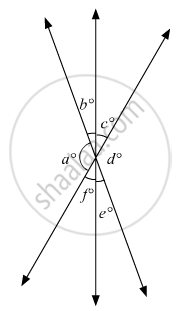•  (i) only

•  (ii) only

•  (iii) only

• (ii) and (iii) only

Q 10 | Page 53

If two interior angles on the same side of a transversal intersecting two parallel lines are in the ratio 2:3, then the measure of the larger angle is

•  54°

•  120°

•  108°

•  136°

Q 11 | Page 53

In the given figure, if AB || CD, then the value of x is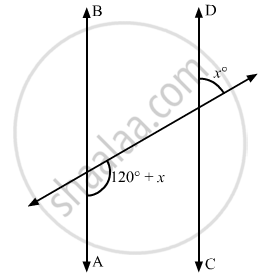• 20°

• 30°

• 45°

• 60°

Q 12 | Page 53

Two lines AB and CD intersect at O. If ∠AOC + ∠COB + ∠BOD = 270°, then ∠AOC =

• 70°

• 80°

• 90°

• 180°

Q 13 | Page 53

In the given figure, PQ || RS, ∠AEF = 95°, ∠BHS = 110° and ∠ABC = x°. Then the value of x is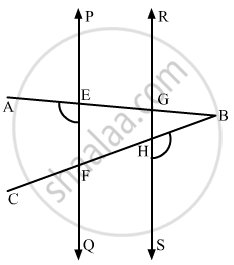•  15°

• 25°

• 70°

• 35°

Q 14 | Page 53

In the given figure, if l1 || l2, what is the value of x?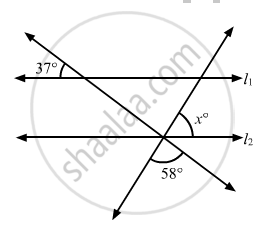• 80°

• 85°

• 90°

• 95°

Q 15 | Page 54

In the given figure, if l1 || l2, what is x + y in terms of w and z?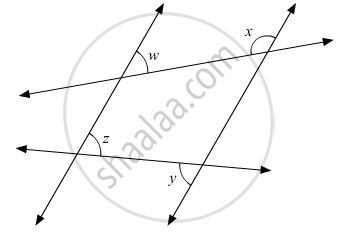• 180° − w + z

•  180° + w − z

•  180° - w − z

•  180° + wz

Q 16 | Page 54

In the given figure, if l1 || l2, what is the value of y?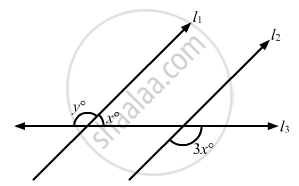• 100°

• 120°

• 135°

•  150°

Q 17 | Page 54

In the given figure, if l1 || l2 and l3 || l4, what is y in terms of x?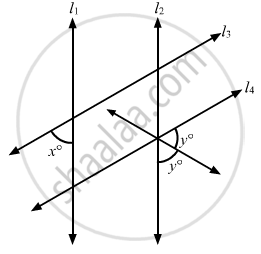•  90 + x

•  90 + 2x

•  90 + x/2

• 90 − 2x

Q 18 | Page 55

In the given figure, if l || m, what is the value of x?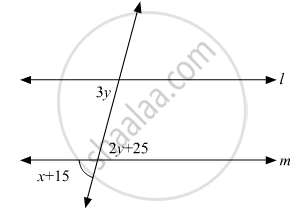• 60°

• 50°

• 45°

• 30°

Q 19 | Page 55

In the given figure, if AB || HF and DE || FG, then the measure of ∠FDE is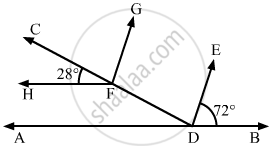• 108°

• 80°

• 100°

• 90°

Q 20 | Page 55

In the given figure, if lines l and m are parallel, then x =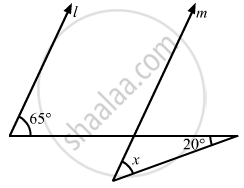• 20°

• 45°

• 65°

• 85°

Q 21 | Page 55

In the given figure, if AB || CD, then x =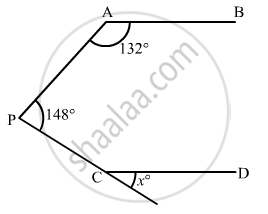• 100°

• 105°

• 110°

• 115°

Q 22 | Page 56

In the given figure, if lines l and m are parallel lines, then x =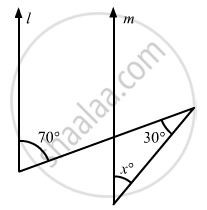• 70°

• 100°

• 40°

• 30°

Q 23 | Page 56

In the given figure, if l || m, then x =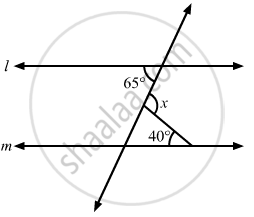• 105°

• 65°

• 40°

• 25°

Q 24 | Page 56

In the given figure, if lines l and m are parallel, then the value of x is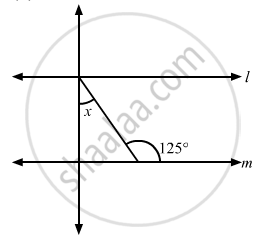• 35°

• 55°

• 65°

• 75°

Q 25 | Page 56

Two complementary angles are such that two times the measure of one is equal to three times the measure of the other. The measure of the smaller angle is

• 45°

• 30°

• 36°

•  none of these

Q 26 | Page 57

In the given figure, if $\frac{y}{x} = 5$ and $\frac{z}{x} = 4$ then the value of x isc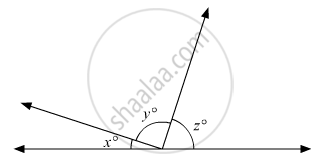•  8°

•  18°

•  12°

•  15°

Q 27 | Page 57

In the given figure, AB || CD || EF and GH || KL. The measure of ∠HKL is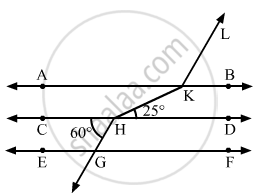• 85°

• 135°

• 145°

• 215°

Q 28 | Page 57

AB and CD are two parallel lines. PQ cuts AB and CD at E and F respectively. EL is the bisector of ∠FEB. If ∠LEB = 35°, then ∠CFQ will be

• 55°

• 70°

• 110°

• 130°

Q 29 | Page 57

In the given figure, If line segment AB is parallel to the line segment CD, what is the value of y?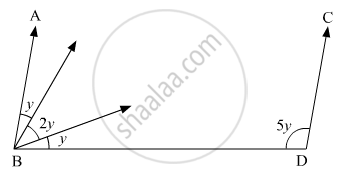• 12

• 15

• 18

• 20

Q 30 | Page 57

In the given figure, if CP || DQ, then the measure of x is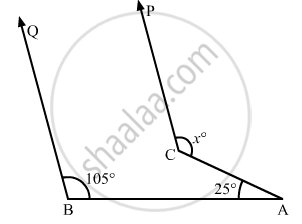• 130°

•  105°

• 175°

• 125°

## Chapter 10: Lines and Angles

Ex. 10.1Ex. 10.2Ex. 10.3Ex. 10.4Others## RD Sharma solutions for Mathematics for Class 9 chapter 10 - Lines and Angles

RD Sharma solutions for Mathematics for Class 9 chapter 10 (Lines and Angles) include all questions with solution and detail explanation. This will clear students doubts about any question and improve application skills while preparing for board exams. The detailed, step-by-step solutions will help you understand the concepts better and clear your confusions, if any. Shaalaa.com has the CBSE Mathematics for Class 9 solutions in a manner that help students grasp basic concepts better and faster.

Further, we at Shaalaa.com provide such solutions so that students can prepare for written exams. RD Sharma textbook solutions can be a core help for self-study and acts as a perfect self-help guidance for students.

Concepts covered in Mathematics for Class 9 chapter 10 Lines and Angles are Concept to Lines and Angles, Basic Terms and Definitions, Intersecting Lines and Non-intersecting Lines, Pairs of Angles, Parallel Lines and a Transversal, Lines Parallel to the Same Line, Angle Sum Property of a Triangle.

Using RD Sharma Class 9 solutions Lines and Angles exercise by students are an easy way to prepare for the exams, as they involve solutions arranged chapter-wise also page wise. The questions involved in RD Sharma Solutions are important questions that can be asked in the final exam. Maximum students of CBSE Class 9 prefer RD Sharma Textbook Solutions to score more in exam.

Get the free view of chapter 10 Lines and Angles Class 9 extra questions for Mathematics for Class 9 and can use Shaalaa.com to keep it handy for your exam preparation

S